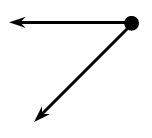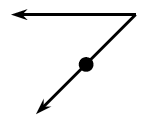### Home > INT2 > Chapter 3 > Lesson 3.2.1 > Problem3-77

3-77.

Juan decided to test what would happen if he rotated an angle.

Copy the angle on your paper and do what Juan does.

1. He copied the angle at right on tracing paper and rotated it $180^\circ$ about its vertex. What type of angle pair did he create? What is the relationship of these angles?

He created a vertical angle pair. The angles have equal measures.2. Juan then rotated the same angle $180^\circ$ through a different point (see the diagram at right). On your paper, draw Juan’s angle and the rotated image. Describe the overall shape formed by the two angles.

They form a letter. Which one?